# final Flashcards

Set Details Share
created 7 years ago by Alissa_Bourgoyne
1,062 views
updated 6 years ago by Alissa_Bourgoyne
Page to share:
Embed this setcancel
COPY
code changes based on your size selection
Size:
X

1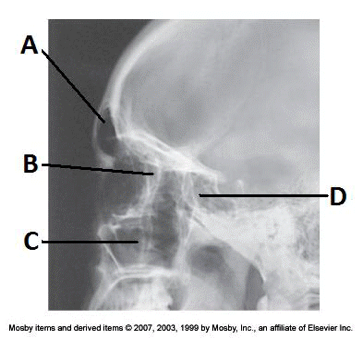4. Examine the image of the paranasal sinuses below. The letter D labels the:

a. maxillary sinuses

b. ethmoid sinuses

c. sphenoid sinuses

d. frontal sinuses

c. sphenoid sinuses

2

____ 5. How many bones comprise the bony orbit?

a. 11

b. 7

c. 9

d. 5

bb. 7

3

____ 6. The parietoacanthial projection (Waters method) of the sinuses requires the orbitomeatal line to be placed how many degrees from the plane of the IR?

a. 30 degrees

b. 20 degrees

c. 27 degrees

d. 37 degrees

dd. 37 degrees

4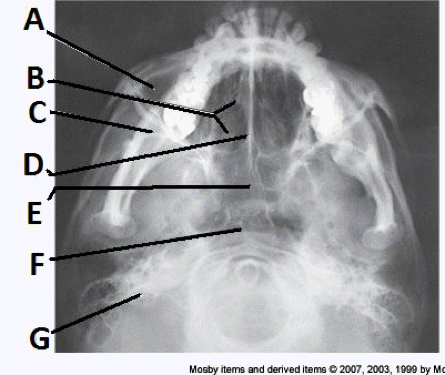__ 7. Examine the image of the paranasal sinuses below. The letter B labels the:

a. ethmoid sinuses

b. sphenoid sinuses

c. maxillary sinuses

d. frontal sinuses

a. ethmoid sinuses

5

____ 9. The OML forms an angle of how many degrees from the plane of the IR for an open-mouth parietoacanthial (Waters method) projection?

a. 45 degrees

b. 25 degrees

c. 35 to 40 degrees

d. 37 degrees

dd. 37 degrees

6____ 10. Examine the image of the paranasal sinuses below. The letter D labels the:

a. crista galli

b. vomer

c. cribriform plate

d. perpendicular plate of the ethmoid bone

bb. vomer

7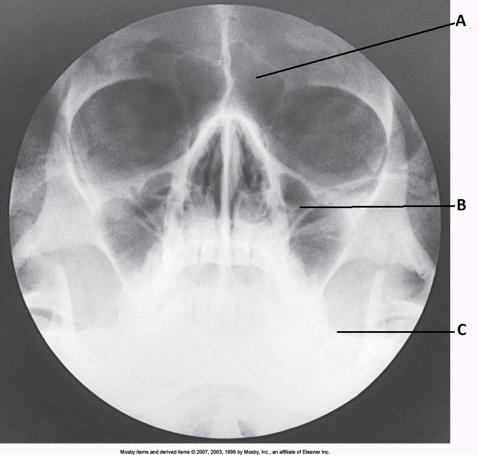____ 11. Examine the image of the paranasal sinuses below. What projection (method) is demonstrated?

a. PA axial (Caldwell)

b. parietoacanthial (Waters)

c. SMV

d. lateral

b

b. parietoacanthial (Waters)

8

____ 12. The two ethmoidal sinuses are located within which bone?

a. maxillary

b. sphenoid

c. frontal

d. ethmoid

dd. ethmoid

9____ 13. Examine the image of the paranasal sinuses below. The letter A labels the:

a. sphenoid sinuses

b. frontal sinuses

c. ethmoid sinuses

d. maxillary sinuses

bb. frontal sinuses

10

____ 185. Which reference line is positioned perpendicular to the angled IR for the PA axial (Caldwell method) projection of the sinuses?

a. AML

b. OML

c. MML

d. IOML

bb. OML

11

____ 23. Which of the following foramina will be demonstrated in the orbit on a parietoacanthial (Waters method) radiograph?

a. ovale

b. spinosum

c. jugular

d. rotundum

dd. rotundum

12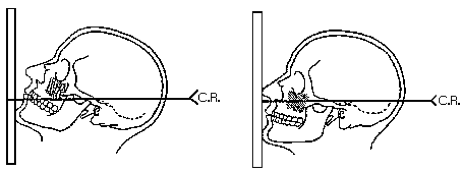____ 30. Which drawing in the figure below demonstrates the correct head position for the Waters method?

a. left

b. right

aa. left

13

____ 31. Which of the following are included as functions of the sinuses?

(1) decrease the weight of the skull

(2) warm and moisten inhaled air

(3) provide a resonating chamber for voice

a. 1 and 2

b. 1 and 3

c. 1, 2, and 3

d. 2 and 3

cc. 1, 2, and 3

14____ 32. Examine the image of the paranasal sinuses below. The letter A labels the:

a. sphenoid sinuses

b. frontal sinuses

c. crista galli

d. ethmoid sinuses

bb. frontal sinuses

15____ 37. Examine the image of the paranasal sinuses below. The letter F labels the:

a. maxillary sinuses

b. sphenoid sinuses

c. ethmoid sinuses

d. pharynx

dd. pharynx

16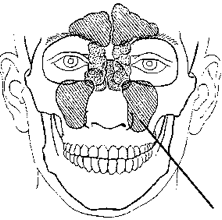____ 43. The sinus identified in the figure below is the:

a. ethmoidal

b. frontal

c. sphenoidal

d. maxillary

dd. maxillary

17

____ 44. The central ray forms an angle of how many degrees with the OML for the PA axial (Caldwell) projection of the sinuses?

a. 12 degrees

b. 15 degrees

c. 5 degrees

d. 20 degrees

bb. 15 degrees

18

____ 46. Which of the following reference lines is placed perpendicular to the IR for a parietoacanthial (Waters method) projection?

a. OML

b. AML

c. IOML

d. MML

dd. MML

19

____ 50. Which sinus is projected through the mouth on the open-mouth modification of the Waters method?

a. maxillary

b. sphenoidal

c. frontal

d. ethmoidal

b. sphenoidal

20

____ 56. At what age are all of the sinuses completely developed?

a. 18

b. 10

c. 8

d. 14

aa. 18

21

____ 60. Which of the following should be clearly demonstrated on a lateral projection of the paranasal sinus?

(1) all four sinus groups

(2) superimposed orbital roofs

(3) superimposed mandibular rami

a. 2 and 3

b. 1, 2, and 3

c. 1 and 3

d. 1 and 2

bb. 1, 2, and 3

22____ 61. Examine the image of the paranasal sinuses below. The letter C labels the:

a. petrous ridge

b. vomer

c. sphenoid sinuses

d. condyle of the mandible

aa. petrous ridge

23

____ 62. Which of the following must be projected below the maxillary sinuses for the parietoacanthial projection (Waters method) of the sinuses?

a. sphenoidal sinuses

b. petrous pyramids

c. inferior orbital margin

d. zygomatic bones

bb. petrous pyramids

24

____ 168. What projection (method) of the facial bones is demonstrated in the image below?

a. AP axial (Towne)

b. PA axial (Caldwell)

c. parietoacanthial (modified Waters)

d. parietoacanthial (Waters)

delete

cc. parietoacanthial (modified Waters)

25

____ 79. The most effective way to protect the patient from unnecessary radiation during sinus radiography is to use:

(1) low kVp

(2) proper collimation

(3) short exposure times

a. 3 only

b. 1 only

c. 1, 2, and 3

d. 2 only

dd. 2 only

26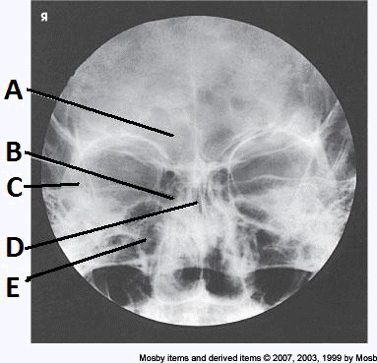____ 84. Examine the image of the paranasal sinuses below. The letter A labels the:

a. maxillary sinuses

b. ethmoid sinuses

c. sphenoid sinuses

d. frontal sinuses

dd. frontal sinuses

27

____ 101. Which of the sinuses is developed at birth and visible radiographically?

a. maxillary

b. sphenoidal

c. frontal

d. ethmoidal

aa. maxillary

28

____ 192. To successfully demonstrate the ethmoidal and sphenoidal sinuses on the SMV projection, which of the following must occur?

(1) the patient must be recumbent

(2) the infraorbitomeatal line must be parallel with the IR

(3) the central ray must be perpendicular to the infraorbitomeatal line and horizontal

a. 2 and 3

b. 1 and 2

c. 1 and 3

d. 1, 2, and 3

aa. 2 and 3

29

____ 112. Which projection will best demonstrate the frontal and anterior ethmoidal sinuses?

(1) PA

(2) parietoacanthial (Waters)

(3) PA axial (Caldwell)

a. 1 only

b. 2 only

c. 3 only

d. 1, 2, and 3

c

c. 3 only

30

____ 125. Where are the petrous ridges seen on a parietoacanthial (Waters method) radiograph?

a. in the lower two thirds of the maxillary sinuses

b. inferior to the floor of the maxillary sinuses

c. superior to the maxillary sinuses

d. in the middle of the maxillary sinuses

bb. inferior to the floor of the maxillary sinuses

31

____ 127. Which sinus is located immediately below the sella turcica?

a. maxillary

b. ethmoidal

c. sphenoidal

d. frontal

cc. sphenoidal

32

____ 129. For the open-mouth modification of the Waters method, the central ray should exit the:

a. acanthion

b. glabella

c. nasion

d. open mouth

dd. open mouth

33

____ 130. Patients who are having an examination of the paranasal sinuses should always be examined in the upright position to:

(1) demonstrate the presence or absence of fluid

(2) differentiate between fluid and other pathological conditions

(3) make it easier for the radiographer to position the patient

a. 1 and 2

b. 2 and 3

c. 1 and 3

d. 1, 2, and 3

aa. 1 and 2

34____ 136. Examine the image of the paranasal sinuses below. The letter B labels the:

a. frontal sinuses

b. maxillary sinuses

c. ethmoid sinuses

d. sphenoid sinuses

cc. ethmoid sinuses

35

____ 137. The respiration phase for all projections of the facial bones and sinuses is:

a. inspiration

b. shallow breathing

c. suspended

d. expiration

c. suspended

36

___ 142. Which reference line is positioned horizontal to ensure proper extension of the head during a lateral projection of the sinuses?

a. MML

b. AML

c. OML

d. IOML

dd. IOML

37

____ 148. Which projections will demonstrate the ethmoidal sinuses?

(1) lateral

(2) PA axial (Caldwell)

(3) SMV

a. 1 and 2

b. 2 and 3

c. 1, 2, and 3

d. 1 and 3

cc. 1, 2, and 3

38

____ 159. The largest sinus is the:

a. frontal

b. maxillary

c. sphenoidal

d. ethmoidal

bb. maxillary

39

____ 165. The central ray is directed perpendicular to which reference line for the SMV projection of the sinuses?

a. OIML

b. MML

c. AML

d. OML

aa. OIML

40

____ 168. What projection (method) of the facial bones is demonstrated in the image below?

a. AP axial (Towne)

b. PA axial (Caldwell)

c. parietoacanthial (modified Waters)

d. parietoacanthial (Waters)

cc. parietoacanthial (modified Waters)

41____ 174. Examine the image of the paranasal sinuses below. The letter E labels the:

a. sphenoid sinuses

b. ethmoid sinuses

c. frontal sinuses

d. maxillary sinuses

aa. sphenoid sinuses

42

____ 177. When using the angled grid technique for the PA axial (Caldwell method) projection of the sinuses, the vertical grid device must be angled:

a. 20 degrees

b. 7 degrees

c. 15 degrees

d. 10 degrees

cc. 15 degrees

43

____ 179. At which level should the central ray enter the base of the skull for the SMV projection of the sinuses?

a. inch anterior to the level of the EAM

b. 1 inch below the mental protuberance

c. inch below the mental protuberance

d. inch posterior to the level of the EAM

aa. inch anterior to the level of the EAM

44

____ 182. Where is the IR centered for the parietoacanthial (Waters method) projection of the sinuses?

a. nasion

b. acanthion

c. inion

d. glabella

bb. acanthion

45____ 184. Examine the image of the paranasal sinuses below. The letter B labels the:

a. maxillary sinuses

b. sphenoid sinuses

c. frontal sinuses

d. ethmoid sinuses

aa. maxillary sinuses

46

____ 185. Which reference line is positioned perpendicular to the angled IR for the PA axial (Caldwell method) projection of the sinuses?

a. AML

b. OML

c. MML

d. IOML

bb. OML

47

____ 187. Which of the following projections will best demonstrate the maxillary sinuses?

(1) parietoacanthial (Waters)

(2) PA axial (Caldwell)

(3) submentovertical

a. 2 only

b. 3 only

c. 1 only

d. 1, 2, and 3

cc. 1 only

48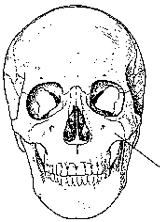____ 188. The bone identified in the figure below is the:

a. maxilla

b. ethmoid

c. frontal

d. mandible

aa. maxilla

49____ 191. Examine the image of the paranasal sinuses below. The letter C labels the:

a. ethmoid sinuses

b. maxillary sinuses

c. petrous ridge

d. sphenoid sinuses

bb. maxillary sinuses

50

Which of the following statements is (are) true regarding a PA Axial projection of the paranasal sinuses?

1. the OML is elevated 15 degrees from the horizontal

2. the petrous pyramids completely fill the orbits

3. the frontal and ethmoidal sinuses are visualized

a. 1 only

b. 1 and 2 only

c. 1 and 3 only

d. 1, 2, and 3

c. 1 and 3 only

51

inflammation of one or more of the paranasal sinuses describes

a. parasitis

b. sinusitis

c. nasalitis

d. mucusitis

b. sinusitis